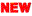All types of recruitment information and pdf material are considered. Special news for teachers, teachers need to have the necessary educational materials. gujarat information site.

Hello friends On this website you will be provided all information like primary education, competitive exams and current trends. Keep visiting our website regularly. thanks for visit www.studymaterials.xyz

EXAM: UNIT TEST IN GUJARAT PRIMARY SCHOOL
MARKS: 25
SUBJECT: MATHS
DATE: 28/12/2019
STD: 4

EKAM KASOTI STD 4 MATHS UNIT TEST PAPER SOLUTION GANIT DATE 28/12/2019
Uses the basic functions of numbers in everyday life.
Has an understanding of numbers up to 10000.
Rounds up to 10000 numbers.
Writes up to 10000 words in words.
Specifies the location value up to 10000.

Multiplies the number of one digits by the number of one digits.

Multiplies a two-digit number with a two-digit number.

Multiplies the one digit number by three digit number.

Solves sum-based practical puzzles.

Subtraction solves practical puzzles.

Solve multiplication-based practical puzzles.

Solve division-based practical puzzles.

Creates his own accounting routine.

The currency sums up to 1000 on a note-coin basis.

Solves practical puzzles based on clocks and divisions.

One number is divided by another, by the interrelationships of various methods, such as graphical, homogeneous groups, repeated subtraction, multiplication, and division.
unit test papers solution semester 2 primary school 2019

1.  ekam kasoti (30/11/19) Paper Solution std 3to 8
3.  ekam kasoti (07/12/19) Paper Solution std 3to 8
7.  ekam kasoti (21/12/19) Paper Solution

1.kasotinu dhora pramane alag Timetable
2.Kasoti New Gun Patrak (Unit test Marksheet) pdf CLICK HERE

Understands numerical patterns and transcends them.

Works with fractions.

Understands fractions as part of an object.

Uses fractions in everyday life.

Identifies one-third, one-fourth, three-quarters of the given pictures.

Displays fractions such as half, points, pa, with symbols like 1/2, 3/4, 1/4.

Shows similarities between 1/2 and 2/4 and other fractions.

Has an understanding of the shapes found in the surrounding environment.

Identifies different patterns of geometric shapes.

Creates a mirror diagram to understand the concept of the convention based on paper twisting or fingerprints.

Understands its appearance by looking at things from a different perspective.

Draws things into perspective.

Answers questions about spatial understanding and directions based on a given map.

Understand the determination of the center, radius and diameter of the circle. Identifies the center, radius, and diameter of a circle.
Indicates the center, radius, and diameter of the circle.
Draws a circle using a circle.
Draws a variety of circle based patterns.
Finds and creates shapes (ladi) needed to create floor patterns.

Given a given shape as a unit, represents the perimeter and area of ​​the geometric shapes (triangles, rectangles, squares). E.g. The notebook states the number of notebooks needed to cover the table headings.